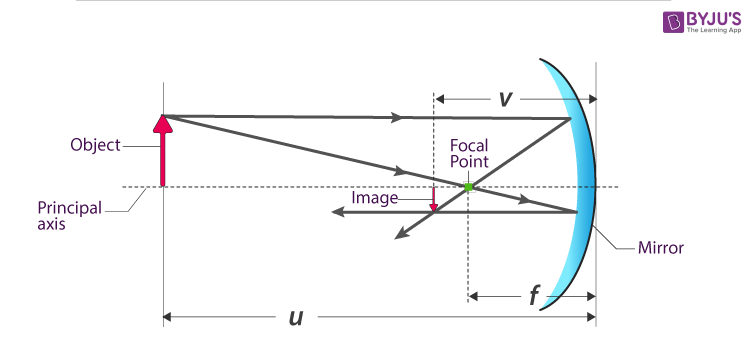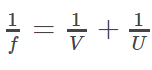# Mirror Formula MCQs

An equation relating object distance and image distance with focal length is known as a mirror equation. It is also known as mirror formula.• The distance between the object and the pole of the mirror is called the object distance (u).
• The distance between the image and the pole of the mirror is called Image distance (v).
• The distance between the Principal focus and pole of the mirror is called Focal Length (f).

The following assumptions are taken in order to derive the mirror formula.

• The distances are being measured from the pole of the mirror.
• According to the convention, the negative sign indicates the distance measured in the direction opposite to the incident ray while the positive sign indicates the distance measured in the direction of the incident ray.
• The distance below the axis is negative whereas the distance above is positive.

Q1: A convex mirror of focal length f forms an image which is 1/n times the object. The distance of the object from the mirror is

1. (n-1)f
2. (n-1/n) f
3. (n+1/n) f
4. (n+1) f

Q2: Consider a concave mirror of focal length 50 cm. Where should the object be placed so that its image is twice the size of the object and inverted

1. 60 cm
2. 70 cm
3. 75 cm
4. 55 cm

Q3: An object 2 cm high is placed at a distance of 16 cm from a concave mirror, which produces 3 cm high inverted image. What is the focal length of the mirror?

1. -10 cm
2. -9.6 cm
3. 9.6 cm
4. 8 cm

Q4: An erect image 3 times the size of the object is obtained with a concave mirror of radius of curvature 36 cm. What is the position of the object?

1. -12 cm
2. 5 cm
3. 10 cm
4. -21 cm

Q5: An object is placed at infinity in front of the convex mirror of radius of curvature 40 cm. How far is the image behind the mirror?

1. 20 cm
2. 25 cm
3. 30 cm
4. 35 cm

Q6: The image formed by a convex mirror of focal length 30cm is a quarter of the object. What is the distance of the object from the mirror?

1. -90 cm
2. 60 cm
3. -75 cm
4. -60 cm

Q7: Convex mirror is used to form an image of the object. Which of the following statements is wrong?

1. The image is erect
2. The image is diminished in size
3. The image is real
4. The image lies between the pole and the focus

Answer: (c) The image is real

Q8: A lens having a focal distance of 30 cm is placed in front of an object, which is located at 1m from it. Where is an image of the object located?

1. 40 cm
2. 42.85 cm
3. 52 cm
4. 60 cm

Q9: The focal length of a concave mirror is f and the distance from the object to the principle focus is x. The ratio of the size of the image to the size of the object is

1. (f + x)/f
2. f/x
3. f2/x2

Q10: In a concave mirror experiment, an object is placed at a distance x1 from the focus and the image is formed at a distance xfrom the focus. The focal length of the mirror would be

1. x1x2
2. (x1+x2)/2
3. √x1x2
4. √x1/x2